# Class 8 Maths NCERT Solutions for Chapter – 8 Comparing Quantities Ex – 8.2

## Comparing Quantities

Question 1.
A man got a 10% increase in his salary. If his new salary is ₹ 1,54,000, find his original salary.

Solution:
Let the salary before increment be ₹ 100. Then,
Increase in salary = ₹ 10
∴ Increased salary = ₹ (100 +10) = ₹ 110
If increased salary is ₹ 110, original salary = ₹100
If increased salary is ₹ 154000, original salary
= ₹$\left( \frac { 100 }{ 110 } \times 154000 \right)$
= ₹ 14000
Hence, the salary of the man before increment was ₹ 140000.

Question 2.
On Sunday 845 people went to the Zoo. On Monday only 169 people went. What is the per cent decrease in the people visiting the Zoo on Monday?

Solution:
On Sunday the number of people went to Zoo = 845
On Monday the number of people went to Zoo = 169
∴ Decrease in the people visiting the Zoo = (845-169) =676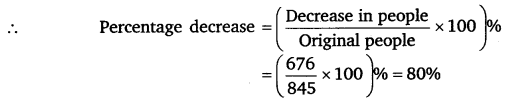Question 3.
A shopkeeper buys 80 articles for ₹ 2,400 and sells them for a profit of 16%. Find the selling price of one article.

Solution:
CP of 80 articles = ₹ 24000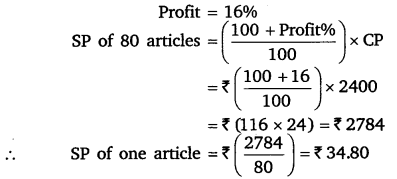Question 4.
The cost of an article is ₹ 15,500. ₹ 450 were spent on its repairs. If it is sold for a profit of 15%, find the selling price of the article.

Solution:
The effective cost price of the article is equal to the price at which it was bought plus the repair cost.
∴ CP of the given article = ₹ (15500 + 450) = ₹ 15950
Profit =15%
∴ Profit =15% of ₹ 15950
= ₹$\left( \frac { 15 }{ 100 } \times 15950 \right)$ = ₹ 2392.50
∴ SP = CP + Profit
= ₹ (15950 + 2392.50) = ₹ 18342.50

Question 5.
A VCR and TV were bought for ? 8,000 each. The shopkeeper made a loss of 4% on the VCR and a profit of 8% on the TV. Find the gain or loss per cent on the whole transaction.

Solution:
For VCR, we have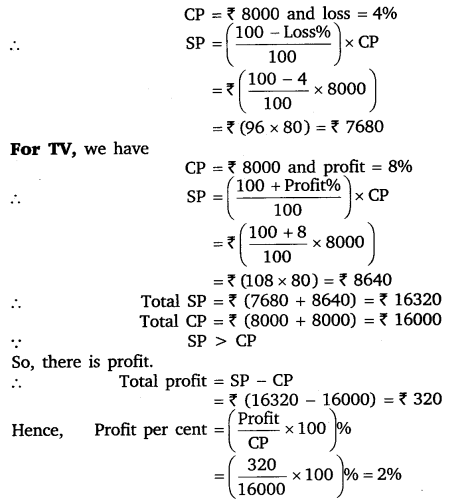Question 6.
During a sale, a shop offered a discount of 10% on the marked prices of all the items. What would a customer have to pay for a pair of jeans marked at ? 1450 and two shirts marked at ? 850 each?

Solution:
For a pair of jeans, we haveQuestion 7.
A milkman sold two of his buffaloes for ? 20,000 each. On one he made a gain of 5% and on the other a loss of 10%. Find his overall gain or loss.

Solution:
For the first buffalo, we have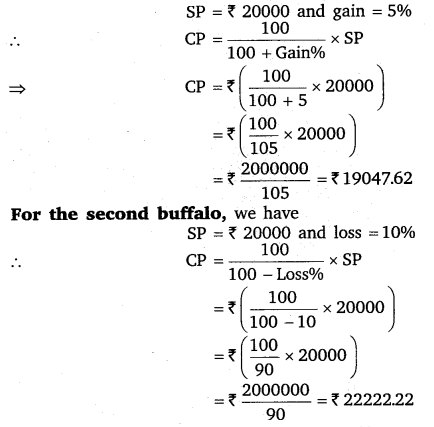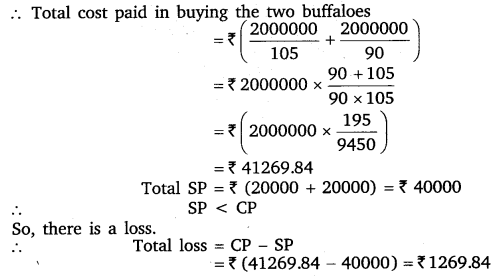Question 8.
The price of a TV is 713,000. The sales tax charged on it is at the rate of 12%. Find the amount that Vinod will have to pay if he buys it.

Solution:
List price of TV = ₹ 13000
Rate of sales tax = 12%
∴ Sales tax = 12% of ₹ 13000
= ₹$\left( \frac { 12 }{ 100 } \times 13000 \right)$ = ₹ 1560
So, the total amount which Vinod had to pay for purchasing TV = ₹ (13000 +1560) = ₹ 14560.

Question 9.
Arun bought a pair of skates at a sale where the discount given was 20%. If the amount he pays is 11,600, find the marked price.

Solution:
Let the marked price of a pair of skates be ? 100.
Discount = 20% of MP
= 20% of ₹ 100 = ₹ 20
∴ SP = MP – Discount
= ₹ (100 – 20) = ₹ 80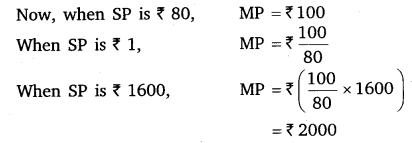Hence, the marked price of the pair of skates is ₹ 2000.

Question 10.
I purchased a hair-dryer for ₹ 5,400 including 8% VAT. Find the price before VAT was added.

Solution:
Let the price of hair-dryer before VAT be ₹ x.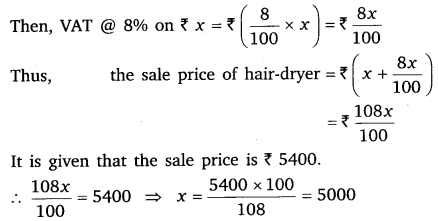Hence, the price of hair-dryer before VAT was ₹ 5000.

#### Class 8 Maths NCERT Solutions for Chapter – 8 Comparing Quantities Ex – 8.3

Lorem ipsum dolor sit amet, consectetur adipiscing elit. Phasellus cursus rutrum est nec suscipit. Ut et ultrices nisi. Vivamus id nisl ligula. Nulla sed iaculis ipsum.

Company Name